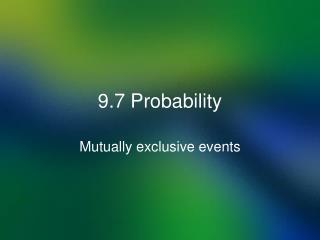# 9.7 Probability - PowerPoint PPT PresentationDownload Presentation9.7 Probability

9.7 ProbabilityDownload Presentation## 9.7 Probability

- - - - - - - - - - - - - - - - - - - - - - - - - - - E N D - - - - - - - - - - - - - - - - - - - - - - - - - - -
##### Presentation Transcript

1. 9.7 Probability Mutually exclusive events

2. Definition of Probability Probability is the Outcomes divided by Sample Space. Outcomes the results of some event. Sample Space is all the possible results of some event. To see this lets look at dice

3. How many different ways can fair 6 sided dice be rolled? Here are the pairs ( first die, second die ) (1,1), (2,1), (3,1), (4,1), (5, 1), (6, 1) (1,2), (2,2), (3,2), (4,2), (5, 2), (6,2) (1,3), (2,3), (3,3), (4,3), (5, 3), (6,3) (1,4), (2,4), (3,4), (4,4), (5, 4), (6,4) (1,5), (2,5), (3,5), (4,5), (5, 5), (6,5) (1,6), (2,6), (3,6), (4,6), (5, 6), (6,6) 36 ways “Sample Space”

4. How many different ways can fair 6 sided dice be rolled to add to 7? Here are the pairs ( first die, second die ) (1,1), (2,1), (3,1), (4,1), (5, 1), (6, 1) (1,2), (2,2), (3,2), (4,2), (5, 2), (6,2) (1,3), (2,3), (3,3), (4,3), (5, 3), (6,3) (1,4), (2,4), (3,4), (4,4), (5, 4), (6,4) (1,5), (2,5), (3,5), (4,5), (5, 5), (6,5) (1,6), (2,6), (3,6), (4,6), (5, 6), (6,6) 6 ways Outcome

5. The Probability of rolling a seven with fair 6 sided dice Using the equation. Outcome is n(E); Sample Space is n(S) Probability is always between 0 and 1. 0 probability never going to happen. 1 probability always going to happen.

6. Mutually Events If you needed to count all the student taking Algebra 2c or Geometry, you would take the class list and add the number of students together. Algebra 2c has 97 students Geometry has 116 students Total 213 students

7. Mutually Events If you needed to count all the student taking Algebra 2c or Geometry, you would take the class list and add the number of students together. Yet you need to remember some students are in both classes, they would need to be subtracted. Students taking both Algebra 2c and Geometry at the same time is 18 students.

8. Mutually Events You need the number students in Algebra 2c or Geometry. Algebra 2c Students 97 Geometry Students 116 Total 213 Students who are taking both classes 18 Subtract Students taking Algebra 2c or Geometry 195

9. Independent Events The Probability of independent events are their individual probabilities multiplied together. What is the possible of rolling a 3 with 3 dice? All the dice would have to be 1. First, second and third dice probability of a one is The probability would be

10. The Complement of an Event Given a probability of something happening, then the probability of something not happening is 100% - the probability. If there was 40 % chance of rain, there is a 60% chance of it not raining.

11. Homework Page 680 – 683 # 4, 8, 12, 26, 36, 46, 58